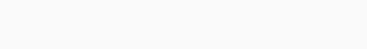# Sports Predictor using Python in Machine Learning

Prediction means to make an estimate of the future and on which base prepare a plan to achieve a goal. Recent technology of computers very useful to predict the future and to make a proper estimate of the event that will happen in the future. The available data, estimate with related connected elements and with the use of computerized techniques with the accurate calculating manner and many others matter keep in mind to predict future events happen. Here we study the Sports Predictor in Python using Machine Learning.

#### Sports Prediction

Prediction also uses for sport prediction. Sports prediction use for predicting score, ranking, winner, etc. There are many sports like cricket, football uses prediction. There technique for sports predictions like probability, regression, neural network, etc. Here we are using sports prediction for cricket using machine learning in Python.

## Building sports predictor in machine learning

Here, We implement a sports predictor in four steps.

Step-1 Importing libraries

Here, we use libraries like Pandas, Numpy, Sklearn.

```import pandas as pd
import numpy as np
import matplotlib.pyplot as plt
import seaborn as sns
from sklearn.model_selection import train_test_split
from sklearn.linear_model import LogisticRegression```

Step-2 Reading and formatting the data

```world_cup=pd.read_csv('World Cup 2019 Dataset.csv')

World_cup.csv has the previous data of all teams.

`world_cup.head()`

Output:results.csv has Previous results between teams.

`result.head()`

Output:Fixtures have the schedule of world cup 2019.

`fixtures.head()`

Output:The ranking file has the current ranking and point of all teams.

```ranking.head()
```

Output:Displaying games played by India.

```india=result[(result['Team_1']=='India')|(result['Team_2']=='India')]

Output:Selecting teams that only participating in the 2019 world cup.

```World_cup_teams=['England', ' South Africa', 'West Indies', 'Pakistan', 'New Zealand', 'Sri Lanka', 'Afghanistan', 'Australia', 'Bangladesh', 'India']
team1=result[result['Team_1'].isin(World_cup_teams)]
team2=result[result['Team_2'].isin(World_cup_teams)]
teams=pd.concat((team1,team2))
teams=teams.drop_duplicates()```

Dropping unnecessary columns.

```team_result=teams.drop(['date','Margin','Ground'],axis=1)

Output:Transforming data into useful information.

```final_result= pd.get_dummies(team_result, prefix=['Team_1', 'Team_2'], columns=['Team_1', 'Team_2'])

Output:Step-3 Building model

Splitting the data into training and testing data.

```X=final_result.drop(['Winner'],axis=1)
y=final_result['Winner']
X_train,X_test,y_train,y_test=train_test_split(X,y,test_size=0.30,random_state=42)```

Initializing model and fitting data into it. Finding scores of train and test data.

```model=LogisticRegression()
model.fit(X_train,y_train)
train_score=model.score(X_train,y_train)
test_score=model.score(X_test,y_test)
print("Traning accuracy: ",train_score)
print("Testing accuracy: ",test_score)```

Output:Step-4 Predicting the winner

For predicting, we make a function. This function can use in any tournament. Here, we use it for world cup 2019. Breaking the function in small to understand function better.

1.  Adding the position of both the team in fixtures.

```fixtures.insert(1,'Team_1_position',fixtures['Team_1'].map(ranking.set_index('Team')['Position']))
fixtures.insert(2,'Team_2_position',fixtures['Team_2'].map(ranking.set_index('Team')['Position']))
fixture=fixtures.iloc[:45,:]

Output:2. Transforming fixture in useful information. Adding some additional columns with value 0.

```final_set=fixture[['Team_1','Team_2']]
final_set = pd.get_dummies(final_set, prefix=['Team_1', 'Team_2'], columns=['Team_1', 'Team_2'])
for col in (set(final_result.columns)-set(final_set.columns)):
final_set[col]=0
final_set=final_set.sort_index(axis=1)
final_set=final_set.drop(['Winner'],axis=1)

Output:3. Predicting the winner.

`prediction=model.predict(final_set)`

4. Displaying the results and storing in the data.

```for index,tuples in fixture.iterrows():
print("Teams: " + tuples['Team_1']+ " and " + tuples['Team_2'])
print("Winner:"+ prediction[index])```

Output:```for i in range(len(prediction)):
fixture['Result'].iloc[i]=prediction[i]```

Plotting the results.

`fixture['Result'].value_counts().plot(kind='bar')`

Output:Combining four parts and making the function.

```def predict_result(matches,final_result,ranking,model,match_type):
#obtaining team position
team_position=[]
for match in matches:
team_position.append([ranking.loc[ranking['Team'] == match,'Position'].iloc,ranking.loc[ranking['Team'] == match,'Position'].iloc])

#transforming data into useful information
final=pd.DataFrame()
final[['Team_1','Team_2']]=pd.DataFrame(matches)
final_set=final
final_set = pd.get_dummies(final_set, prefix=['Team_1', 'Team_2'], columns=['Team_1', 'Team_2'])

for col in (set(final_result.columns)-set(final_set.columns)):
final_set[col]=0
final_set=final_set.sort_index(axis=1)
final_set=final_set.drop(['Winner'],axis=1)

#predict winner
prediction=model.predict(final_set)

#Results from League mathes
if match_type == 'League':
print("League Matches")

final_fixture=fixtures[0:45]
for index,tuples in final_fixture.iterrows():
print("Teams: " + tuples['Team_1']+ " and " + tuples['Team_2'])
print("Winner: "+ prediction[index])
fixtures['Result'].iloc[index]=prediction[index]

Semi_final_teams=[]
for i in range(4):
Semi_final_teams.append(fixture['Result'].value_counts().index[i])
matches=[(Semi_final_teams,Semi_final_teams),(Semi_final_teams,Semi_final_teams)]
match_type="Semi-Final"
predict_result(matches,final_result,ranking,model,match_type)

#Result from semi-final
elif match_type == 'Semi-Final':
print("\nSemi-Final Matches")
final_fixture=fixtures[45:47]
for index,tuples in final_fixture.iterrows():
fixtures['Team_1'].iloc[index]=final['Team_1'].iloc[index-45]
fixtures['Team_2'].iloc[index]=final['Team_2'].iloc[index-45]
fixtures['Team_1_position'].iloc[index]=team_position[index-45]
fixtures['Team_2_position'].iloc[index]=team_position[index-45]
final_fixture=fixtures[45:47]
for index,tuples in final_fixture.iterrows():
print("Teams: " + tuples['Team_1']+ " and " + tuples['Team_2'])
print("Winner: "+ prediction[index-45])
fixtures['Result'].iloc[index]=prediction[index-45]
matches=[(prediction,prediction)]
match_type="Final"
predict_result(matches,final_result,ranking,model,match_type)

#Result of Final
elif match_type == 'Final':
print("\nFinal Match")
final_fixture=fixtures[47:48]
for index,tuples in final_fixture.iterrows():
fixtures['Team_1'].iloc[index]=final['Team_1'].iloc[index-47]
fixtures['Team_2'].iloc[index]=final['Team_2'].iloc[index-47]
fixtures['Team_1_position'].iloc[index]=team_position[index-47]
fixtures['Team_2_position'].iloc[index]=team_position[index-47]
final_fixture=fixtures[47:48]
for index,tuples in final_fixture.iterrows():
print("Teams: " + tuples['Team_1']+ " and " + tuples['Team_2'])
print("Winner: "+ prediction+"\n")
fixtures['Result'].iloc[index]=prediction[index-47]
print("Winner Of the tournament is: " + fixtures['Result'].iloc)```

It can use to predict the following items.

1.  League, semi-final and final matches.

2. the semi-final and final matches.

3. Final matches.

Output:## Dataset for Sports Prediction

The dataset containing four csv file:

fixture.csv

icc_ranking.csv

results.csv

World cup 2019 Dataset.csv

The dataset is available on Kaggle. You can download it from here:  World_cup_2019_Dataset

#### Conclusion

Here, we see the following topics:

• Prediction
• Sport prediction
• Building sports prediction in Python

### 2 responses to “Sports Predictor using Python in Machine Learning”

1. Hamza rafiq says:

Can I run this project on my laptop??if yes!! Then how?

2. Felipe says:

That project is awesome, congrats. I applier for Fifa World Cup 2022 and works.
I understand that I need to collect more games, I used dataset from matches from 1930 to 2018, but more is better for accuracy.
Also I used mean() of the ranking since 1992, I will cut for few years, so the mean will be more accurate as well.